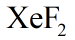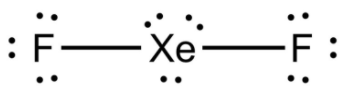xef2 lewis structure homework Help at TutorEye

# Best Homework Help For xef2 lewis structure

## Top Questions

he molarity of the acid solution? H2SO4 + 2 NaOH -> Na2SO4 + 2 H2O
View More

he molarity of the acid solution? H2SO4 + 2 NaOH -> Na2SO4 + 2 H2O
View More

he molarity of the acid solution? H2SO4 + 2 NaOH -> Na2SO4 + 2 H2O
View More

pport if pressure doubles then temp should double as well. 3. What Boyles Law has to do with % range.
View More

##Lewis structure:

Total of 22 valence electrons are present in amolecule, 8 from Xe and 7 from each F. Being a less electronegative atom, Xe is the central atom and forms a single bond with each F atom. Hence, Xe doesn’t follow the octet rule. Each fluorine atom forms a single bond with Xe completing their octet. Each dot represents an electron. A pair of dots or solid line between two atomic symbols represents a bond pair. The pairs of dots on atomic symbols represent lone pairs.##Lewis structure Sample Questions:

Question 1: What is the number of bond pairs inmolecule?

(a) 2

(b) 3

(c) 4

(d) 5

Explanation: Total of 22 valence electrons are present in a  molecule, 8 from Xe and 7 from each F.

Question 2: Number of valence electrons are in?

(a) 6

(b) 10

(c) 16

(d) 22

Explanation: Each F atom has 7 valence electrons. Two F atoms thus have 14 valence electrons.

Question 3: The number of single bonds inis-

(a) 1

(b) 2

(c) 3

(d) 4

Explanation: Total of 22 valence electrons are present in a  molecule, 8 from Xe and 7 from each F.

Question 4: The number of lone pairs inmolecule is?

(a) 0

(b) 3

(c) 6

(d) 9

Explanation:molecule has a total of 22 valence electrons, with 8 from Xe and 7 from each F.

Question 5: How many lone pairs are present on central atom in?

(a) 1

(b) 2

(c) 3

(d) 4

Explanation: A Xenon atom has 8 valence electrons. inmolecule, 2 out of these 8 are used in bonding with two fluorine atoms

Question 6: Why is Xenon central atom in?

(a) It is smaller than fluorine.

(b) It is a less electronegative atom.

(c) It is a more stable atom.

(d) It has more valence electrons.

Explanation: Central atom shares more electrons than a terminal atom.

Question 7: Pick the correct statement about lewis structure of?

(a) It contains two double bonds.

(b) It has 20 valence electrons.

(c) It has 18 nonbonding electrons.

(d) F is the central atom.

Explanation:molecule has a total of 22 valence electrons, with 8 from Xe and 7 from each F.

Question 8: Which atom doesn’t follow the octet rule in Lewis structure of?

(a) Xe

(b) One F

(c) Both F

(d) None of the above

Explanation: Total of 22 valence electrons are present in a.molecule, 8 from Xe and 7 from each F. Being a less electronegative atom.

Question 9: The shape ofmolecule is-

(a) Linear

(b) Bent

(c) Trigonol Planar

(d) Pyramidal

Explanation: Molecular geometry ofis linear.

Question 10: Which of the following statement is incorrect about the Lewis structure of?

(a) Xe is bonded to two F atoms via two single bonds.

(b) Each atom has three lone pairs.

(c) Total number of valence electrons is 22.

(d) All the atoms follow the octet rule.

Explanation: Total of 22 valence electrons are present in amolecule, 8 from Xe and 7 from each F.

### Question 1: What is the XeF2 Lewis structure?

XeF2  has a total of 22 valence electrons, 8 from Xe and 7 from each F. Xe being a less electronegative atom is the central atom and it forms a single bond with each F atom. Therefore, Xe doesn’t follow the octet rule. Each fluorine atom with 7 valence electrons forms a single bond with Xe completing their octet. Each dot represents an electron. A pair of dots between two atomic symbols represents a bond pair. Other pairs of dots on atomic symbols represent lone pairs.Question 2: What is the chemical name for XeF2  ?

XeF2 has xenon as a central atom singly bonded to two fluorine atoms. The name of the compound is Xenon difluoride.

### Question 3: What is the total number of valence electrons in the Lewis structure of XeF2?

Atomic number of Xe is 54 and its Electronic configuration is-

[Kr]5s24d105p6

There are 8 valence electrons in Xe.

Atomic number of F is 9 and its Electronic configuration is-

[He]2s22p5

There are 7 valence electrons in one F atom.

As there is one Xe atom and two F atoms in XeF2 the total number of valence electrons is = 8+(7+7) = 22.

### Question 4: How many lone pairs are there on the lewis structure of XeF2?

XeF2 has a total of 22 valence electrons, 8 from Xe and 7 from each F. Each fluorine atom uses one of the 7 valence electrons to form a single bond with Xe and is left with 6 non-bonding electrons or three lone pairs. Xe uses two of its 8 valence electrons in bonding with 2 F atoms and is left with 6 non-bonding electrons or three lone pairs. Xe and both F have three lone pairs each making total count of lone pairs = 9.